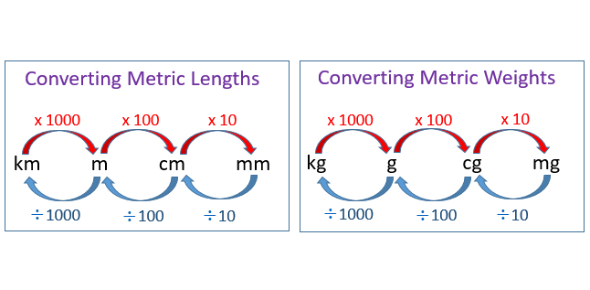# Metric Conversions Knowledge Quiz! Trivia

10 Questions | Attempts: 532
ShareSettingsAre you looking or a metric conversions knowledge quiz trivia? There are different units of measurements which are interrelated all based on what is being measured. One of the simplest ways to understand metric conversions is by using the metric staircase. By using this quiz, you will get to have some much-needed practice. Do give it a shot and keep an eye out for more quizzes just like it!

• 1.
Select the base unit of the metric system for length.
• A.

Liter

• B.

Meter

• C.

Gram

• 2.
Select the base unit of the metric system for capacity (volume).
• A.

Gram

• B.

Liter

• C.

Meter

• 3.
Select the base unit of the metric system for weight (mass).
• A.

Liter

• B.

Meter

• C.

Gram

• 4.
Which prefix means "1000 x (10 x 10 x 10) more than the unit"
• A.

Deci

• B.

Deca

• C.

Kilo

• D.

Centi

• 5.
Which prefix means "10 x less than the unit?"
• A.

Deci

• B.

Centi

• C.

Milli

• D.

Deca

• 6.
Which prefix means "100 (10 x 10) times more than the unit?"
• A.

Deca

• B.

Kilo

• C.

Hecto

• D.

Centi

• 7.
Which prefix means "1000 (10 x 10 x 10) x less than the unit?"
• A.

Milli

• B.

Centi

• C.

Kilo

• D.

Deca

• 8.
If I convert UP the staircase I multiply.
• A.

True

• B.

False

• 9.
If I convert DOWN the staircase I multiply.
• A.

True

• B.

False

• 10.
As I convert up the staircase, the unit gets bigger but the number gets smaller.
• A.

True

• B.

False

## Related TopicsBack to top
×

Wait!
Here's an interesting quiz for you.